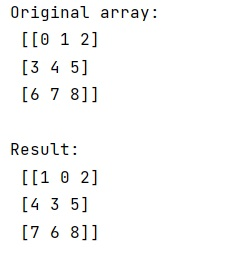# How to swap columns in a numpy array?

Learn, how to swap columns in a numpy array in Python?
Submitted by Pranit Sharma, on January 22, 2023

NumPy is an abbreviated form of Numerical Python. It is used for different types of scientific operations in python. Numpy is a vast library in python which is used for almost every kind of scientific or mathematical operation. It is itself an array which is a collection of various methods and functions for processing the arrays.

## Swapping columns in a numpy array

Suppose that we are given a NumPy array that contains multiple rows and columns, we need to swap two columns with each other.

For this purpose, we have a simple approach of slicing and selecting. We will select our first column by using [: , 0] and the second column by using [: , 1 ] and we will assign their values as the second column and first column respectively.

Let us understand with the help of an example,

## Python code to swap columns in a numpy array

```# Import numpy
import numpy as np

# Creating a numpy array
arr = np.arange(9).reshape(3, 3)

# Display original array
print("Original array:\n",arr,"\n")

# Swapping 1st and second column
arr[:, 0], arr[:, 1] = arr[:, 1], arr[:, 0].copy()

# Display result
print("Result:\n",arr,"\n")
```

Output: